## Example Questions

← Previous 1 3

### Example Question #1 : How To Find Absolute Value

Solve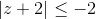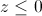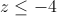All real numbers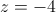No solutions

No solutions

Explanation:

Absolute values measure the distrance from the origin and is always positive, thus it can never be less than or equal to a negative number (unless a negative number is multiplied outside the absolute value).  So the correct answer is no solutions.

### Example Question #2 : How To Find Absolute Value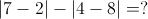Explanation:

Absolute value is the key here. Absolute value means the number's distance from zero. So we must account for that. Therefore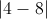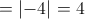.

### Example Question #2 : How To Find Absolute Value

What are the values of a and b, if any, where –a|b + 7| > 0?

a>0 and b not equal to 7

a<0 and b not equal to 7

a>0 and b not equal to 7

a<0 and b = 7

a<0 and b not equal to 7

Explanation:

The absolute value will always yield a positive, as long it is not zero. Therefore, b cannot equal 7. For the value to be positive, a must be a negative number.

### Example Question #4 : How To Find Absolute Value

What is the absolute value of 19 – 36(3) + 2(4 – 87)?

168

–255

–168

293

255

255

Explanation:

19 – 36(3) + 2(4 – 87) =

19 – 108 + 2(–83) =

19 – 108 – 166 = –255

Absolute value is the non-negative value of the expression

### Example Question #5 : How To Find Absolute Value

Solve for z where | z + 1 | < 3

z < 1 or z > 3x

1 < z

1 < z < 3

–4 < z < 2

–4 < z

–4 < z < 2

Explanation:

Absolute value problems generally have two answers:

z + 1 < 3 or z + 1 > –3 and subtracting 1 from each side gives z < 2 or z > –4 which bcomes –4 < z < 2

### Example Question #6 : How To Find Absolute Value

Find the absolute value of the following when x = 2,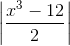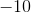Explanation: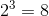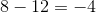and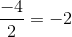It is important to know that the absolute value of something is always positive so the absolute value ofis### Example Question #7 : How To Find Absolute Value

Evaluate for: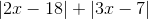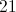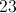Explanation: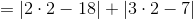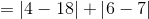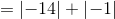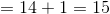### Example Question #8 : How To Find Absolute Value

Evaluate for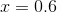: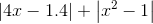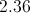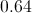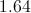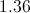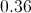Explanation:

Substitute 0.6 for: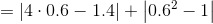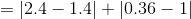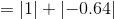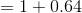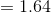### Example Question #9 : How To Find Absolute Value

Evaluate for: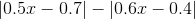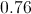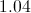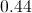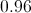Explanation:

Substitute.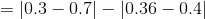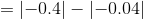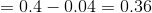### Example Question #10 : How To Find Absolute Value

Which of the following sentences is represented by the equation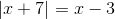The absolute value of the sum of a number and seven is three less than the number.

The absolute value of the sum of a number and seven is three greater than the number.

None of the other responses are correct.

The sum of three and the absolute value of the sum of a number is three less than the number.

The sum of three and the absolute value of the sum of a number is three greater than the number.

The absolute value of the sum of a number and seven is three less than the number.

Explanation: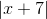is the absolute value of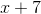, which in turn is the sum of a number and  seven and a number. Therefore,can be written as "the absolute value of the sum of a number and seven". Since it is equal to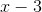, it is three less than the number, so the equation that corresponds to the sentence is

"The absolute value of the sum of a number and seven is three less than the number."

← Previous 1 3

### All ACT Math Resources